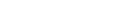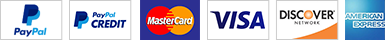### Introduction

DSFT has an extensive range of free-On Line resources for undertaking a wide range of thermal process calculations including dry heat sterilisation (FH) and depyrogenation (FD or FP). This page provides access to a range of Microsoft Excel spreadsheets that can be downloaded for a small donation and will work on a PC or Mac without access to the Internet. In general these spreadsheets are similar to their corresponding free applications on this website.

These donations are important. They contribute towards the running costs of the website and enable me to provide free access to the website rather than require a subscription.

1) A macro-based spreadsheet described similar to the above using the trapezoid rule to determine the lethality (F, value) of a thermal process.

2) An Excel workbook using non-macro based coefficients to implement both of Simpson's rules and the trapezoid rule to calculate F values. This download also contains a spreadsheet to convert Z-values in Fahrenheit or Celsius to the desired measurement system and a PDF document listing D, F, Z values and reference temperatures for the major spoilage organisms of significance to processing low acid and acid foods. A link to a free On Line resource that details thermal resistance data for many spoilage and pathogenic organisms significant to the food and pharmaceutical industries is also provided.

3) A macro based spreadsheet for determining the lethality (F, value) of a thermal process using the trapezoid and Simpson's rules. This is an Excel based version of the calculator at https://www.dairyscience.info/f-s-t/ .

4) A macro based spreadsheet for determining the lethality (F, B* values) and chemical changes (C* value) for UHT processes using the trapezoid and Simpson's rules. This is an Excel based version of the calculator at https://www.dairyscience.info/uht-t/ .

5) A macro based spreadsheet for determining the lethality (F, B* values) and chemical changes (C* value, formation of: HMF, Lactulose, Furosine, and destruction of thiamine) in heated milk integrated using the trapezoid and Simpson's rules. This is an Excel based version of the calculator at https://www.dairyscience.info/uht/ .

6) The author's Ebook "Thermal processing of acid fruit and vegetable products. Significant microorganisms, recommended processing time / temperatures, and public health significance of spoilage".

7) All the spreadsheets above and the author's Ebook "Thermal processing of acid fruit and vegetable products. Significant microorganisms, recommended processing time / temperatures, and public health significance of spoilage."

Purchase can be made securely using major debit or credit cards or PayPal. PayPal processes all financial transactions on this site. Note a PayPal account is not required to make a credit or debit card payment.

### 1)  A macro based spreadsheet using the trapezoid rule to determine the lethality (F, value) of a thermal process. (Trapezoid F macro calculator)Figure 1. Image of the macro-based spreadsheet.

This spreadsheet uses the Microsoft Visual Basic Scripting (VBScript) language to write a script for the trapezoid rule, to validate the inputs (cells must not contain text, be empty and should not contain negative numbers) including the submission of paired sets of data. Errors generate alert messages and individual cells are coloured red to enable easy resolution of input errors. Users also have the option of calculating the number of log reductions of the target microorganism by entering the D-value of the organism and clicking on the calculate button.

By default up to 3000 pairs of time-temperature values can be pasted or typed into the calculator (see Figure 2). The macros are not password protected and you have full access to all the code!Figure 2. Image of the macro-based unrestricted trapesoid spreadsheet### 2) Microsoft Excel workbooks F  programmed using Simpson's rules and the Trapezoid method for comparative analysis. Z value converter included.

While the industry-standard method for obtaining the area under the lethal rate versus time curve is the trapezoid rule, Simpson's rules are more accurate. However, Simpson's rules are somewhat more difficult to implement.

The package below provides users with the tools to study the differences between F values obtained by both methods and to explore using the time-lethal rate plots the effect of the shape of the curve on the differences between both methods.

The workbook contains two worksheets and the details of the trapezoid sheet are shown in (Figure 3).Figure 3. Screen shot of the updated trapezoid spread sheet for calculating F values of thermal processes

Note these spread sheets use advanced Excel programming knowledge and automatically generate data entry grids. Time entry is not required, this is generated automatically once the delta T value has been added. The user only has to paste the temperature values in.

The spreadsheet has been configured to take up to 1400 time-temperature inputs and can be amended to take thousands if required.

A macro-based control has been added to clear temperature values.

The cells coloured yellow are the input cells and the user can set the temperature interval between readings e.g. 0.5° C, the duration of the thermal process, the Z value and the reference temperature. Once the temperature interval and duration of the process are entered a grid will be generated to enable temperature values to be entered; conditional statements are used to generate this grid automatically.

There is also a facility to calculate the number of log reductions of a target microbial group at the reference temperature. To use this facility the user simply enters the corresponding D value at the reference temperature. Note, it is not essential to enter the D-value to calculate the F value. The D value is only required if the user needs to calculate the number of log reductions of a microbial group or organism.

The worksheet programmed using Simpson's rules is shown in Figure 4.Figure 4. Screen shot of the spread sheet programmed using Simpson's rules for calculating F values of thermal processes.

Simpson's one third rule is used to calculate area when the an odd number of data points are used and Simpson's three eights rule is used for the last few points when working with an even number of data points. The spreadsheet undertakes these calculations automatically.

Note this spreadsheet as with the trapezoid based one, does not use a macro to do the complex calculations but uses a series of IF-statements to generate the Simpson's coefficients, 1,2,3 or 4.

Again there is an option to calculate the log reduction in the target microbial group following heat processing.

The spreadsheets were updated (May 2018) to correct a 'bug' on the Simpson's worksheet that can result in incorrect coefficients being generated depending on the decimal fraction used in the time interval. Also a macro-based control to clear all data and reset the spreadsheets has been added.

I frequently get queries asking how to convert Z values from Fahrenheit to Celsius and vice versa and a Z-value converter is also enclosed in the zip file (Figure 5).Figure 5. Screenshot of Z value converter.

A PDF document listing D, F, Z values and reference temperatures for the major spoilage organisms of significance to processing low acid and acid foods is also provided. Additionally a link to a free On Line resource that details thermal resistance data for many spoilage and pathogenic organisms significant to the food and pharmaceutical industries is providedNote all the cell formulas are unlocked. The spreadsheet is for your own personal educational use. The Terms and Conditions that apply to the Ice Cream Mix Spreadsheets also apply.

3) The On Line calculator at https://www.dairyscience.info/f-s-t/ is based on the macro-based spreadsheet for determining the lethality (F, value) of a thermal process using the Trapezoid and Simpson's rules  that can be downloaded from the link below (MBSS). To use you simply paste up to thousands of matched time-temperature values and the Trapezoid and Simpson's macros will calculate F and display the different values of the different calculation methods.

Basic data input validation is also provided.

A screenshot of the spreadsheet is shown in Figure 6 below.Figure 6. Screenshot of macro-based calculator for calculating F using the Trapezoid and Simpson's rules.

Graphs of temperature and lethal rate versus time are plotted and you can choose to select seconds or minutes as the time input units.4) F0, B* and C* are important indicators for measuring the effectiveness of UHT processing.The On Line calculator at https://www.dairyscience.info/uht-t/ is based on the macro-based spreadsheet for determining the lethality (F, value) of a thermal process using the Trapezoid and Simpson's rules that can be downloaded from the link below. To use you simply paste up to thousands of matched time-temperature values and the Trapezoid and Simpsons macros will calculate F0, B* and C* and display the different values obtained using the industry standard method and the more accurate Simpson’s rules.

Basic data input validation is also provided. A screen shot of the spreadsheet is shown in Figure 7 below.Figure 7. Screenshot of macro-based calculator for calculating F0, B* and C* using the Trapezoid and Simpson's rules.

Graphs of temperature and lethal rate versus time are plotted5.) Calculator for determining the lethality (F0, B* values) and chemical changes (C* value, formation of: hydroxymethylfurfural (HMF), lactulose, furosine, and destruction of thiamine) in heated milk integrated using the Trapezoid and Simpson's rules. The On Line calculator at https://www.dairyscience.info/uht/ programmed using the thermal constants calculated by Claeys et al. (2003) to calculate HMF, lactulose and furosine concentrations following heat treatment in skim, semi fat and full fat milks is based on the macro-based spreadsheet that can be downloaded from the link below. To use you click a control box to load default values, select milk type and simply paste up to thousands of matched time-temperature values and the Trapezoid and Simpsons macros will calculate F0, B* and the chemical changes and display the different values obtained using the industry standard method and the more accurate Simpson’s rules. The spreadsheet is provided with basic data input validation and plots time, seconds, versus temperature and lethality.

A screen shot of a portion of the spreadsheet is shown in Figure 8 below. Note you can add your own values for Kref or Ea or simply accept the values of Claeys et al. (2003) by loading the default values.Figure 8. Detail of macro-enabled spreadsheet for calculating lethal values and chemical indicators of UHT processing of milk.

An overview of the complete spreadsheet is given in Figure 9.Figure 9. Screen shot of macro-based spreadsheet for calculating the lethality and chemical changes of UHT-treatment of milk.7.) The author's Ebook "Thermal processing of acid fruit and vegetable products. Significant microorganisms, recommended processing time / temperatures, and public health significance of spoilage"